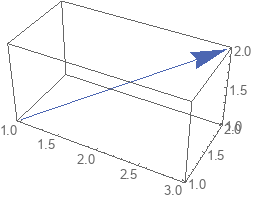#Function Repository Resource:

# PlotVector3D

Plot a list of vectors in space

Contributed by: Dennis M Schneider
 ResourceFunction["PlotVector3D"][{{x1,y1,z1},{x2,y2,z2},…}] plots the vectors {xi,yi,zi}, each located at the origin. ResourceFunction["PlotVector3D"][{{x1,y1,z1},{x2,y2,z2},…},{p,q,r}] plots the vectors {xi,yi,zi}, each located at the point {p,q,r}. ResourceFunction["PlotVector3D"][{{{x1,y1,z1},{p1,q1,r1}},{{x2,y2,z2},{p2,q2,r2}},…}] plots the vectors {xi,yi,zi} located at the corresponding points {pi,qi,ri}. ResourceFunction["PlotVector3D"][{{x1,y1,z1},{x2,y2,z2},…},{{p1,q1,r1},{p2,q2,r2},…}] plots the vectors {xi,yi,zi} located at the corresponding points (pi,qi,r1}.

## Details and Options

The following options are supported:
 "ArrowSize" specifies the size of the arrowhead "LocatedVector" whether to draw vectors from the initial point (the first point of the list) RGBColor[.3,.4,.7] specifes the style of the vector
A located vector is one whose initial point is the first point in the list, and whose terminal point is the second point in the list. ResourceFunction["PlotVector3D"][{{{x,y,z},{p,q,r}}}] uses {p,q,r} as the initial point and moves off in the direction given by {x,y,z}. Thus the terminal point is {p+x,q+y,r+z}.

## Examples

### Basic Examples

Plot the vector (1,1,1) located at the origin:

 In:=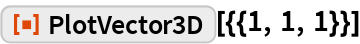Out=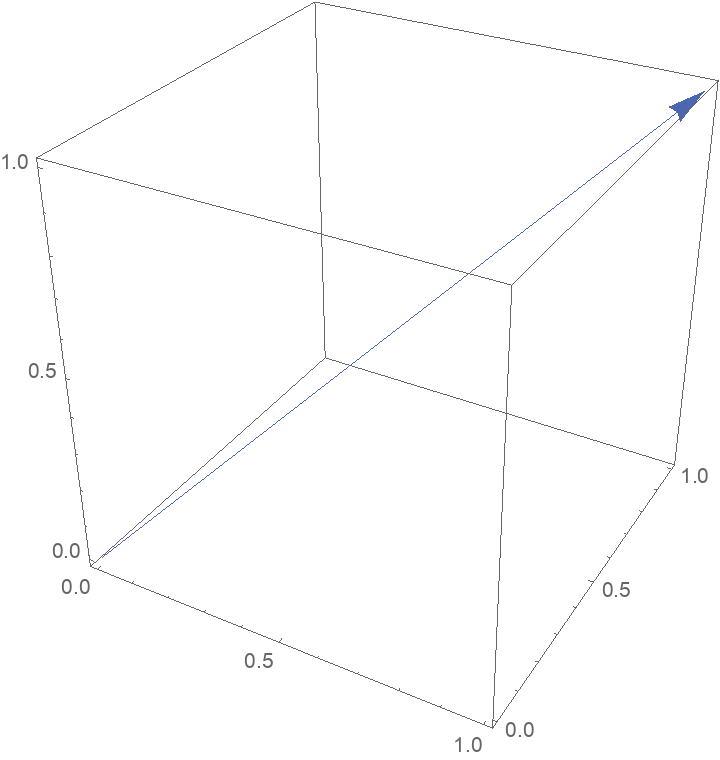Plot a list of vectors located at the origin:

 In:=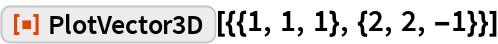Out=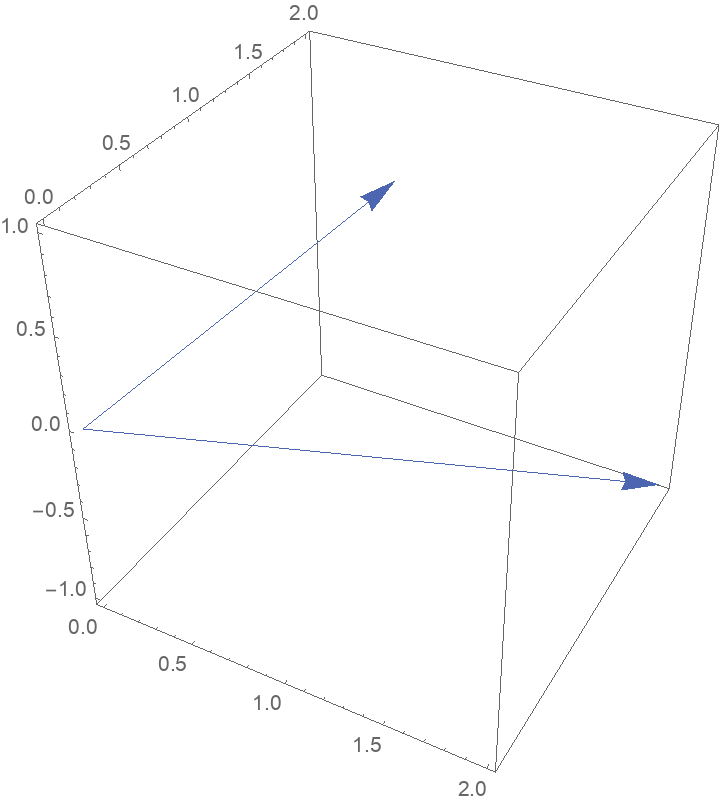### Scope

Plot the vector (2,2,2) located at the point (-1,1,1):

 In:=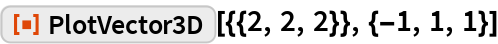Out=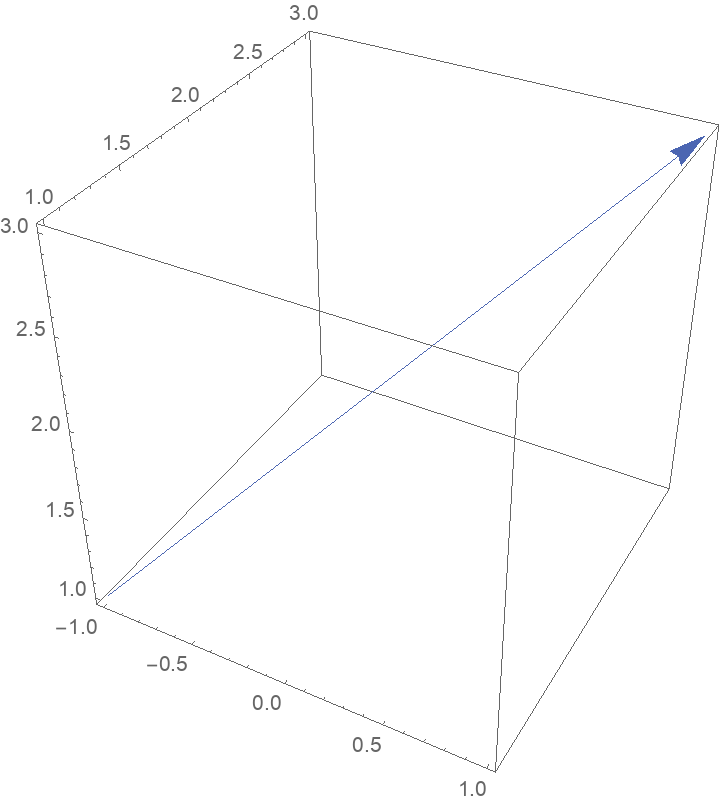Plot a list of vectors located at the point {1,2,2}:

 In:=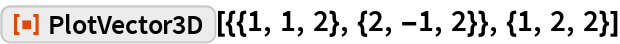Out=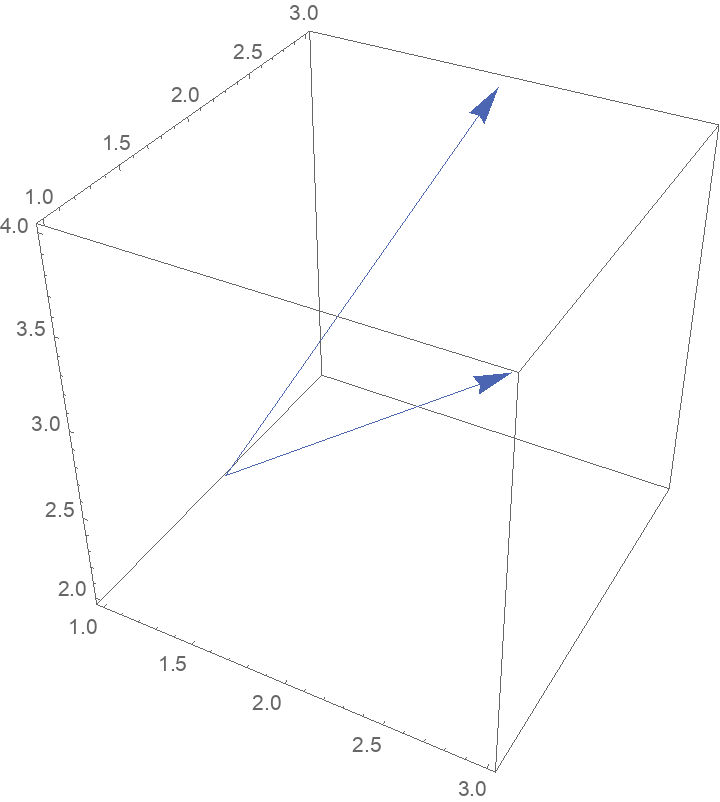Plot a list of vectors located at different points. The first list is a list of vectors and the second is a list of initial points:

 In:=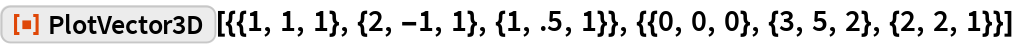Out=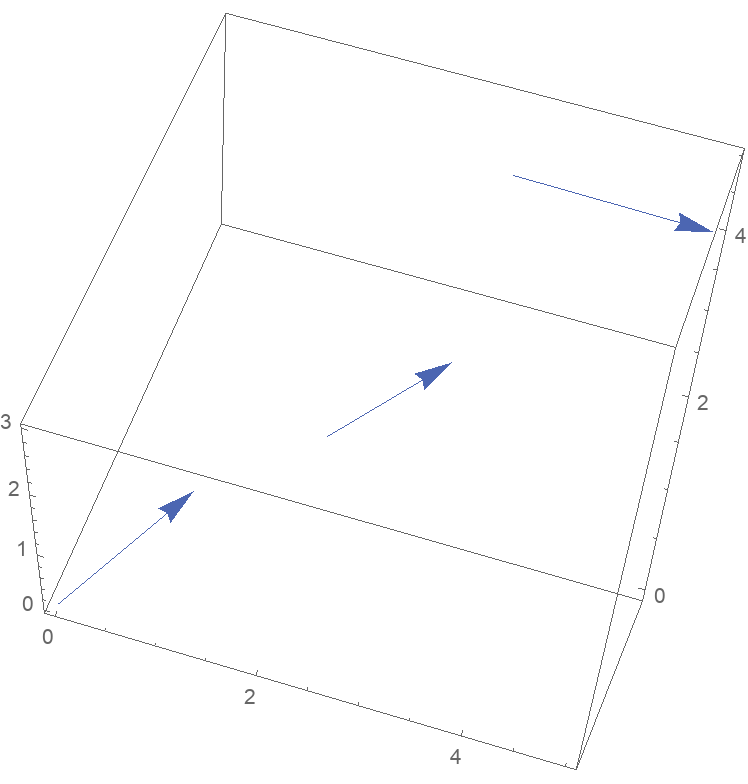The length of the list of vectors must be the same as the length of the list of initial points:

 In:=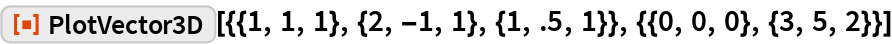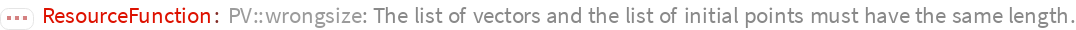### Options

#### ArrowSize

Change the arrow size of each vector:

 In:=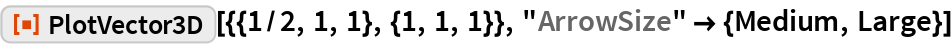Out=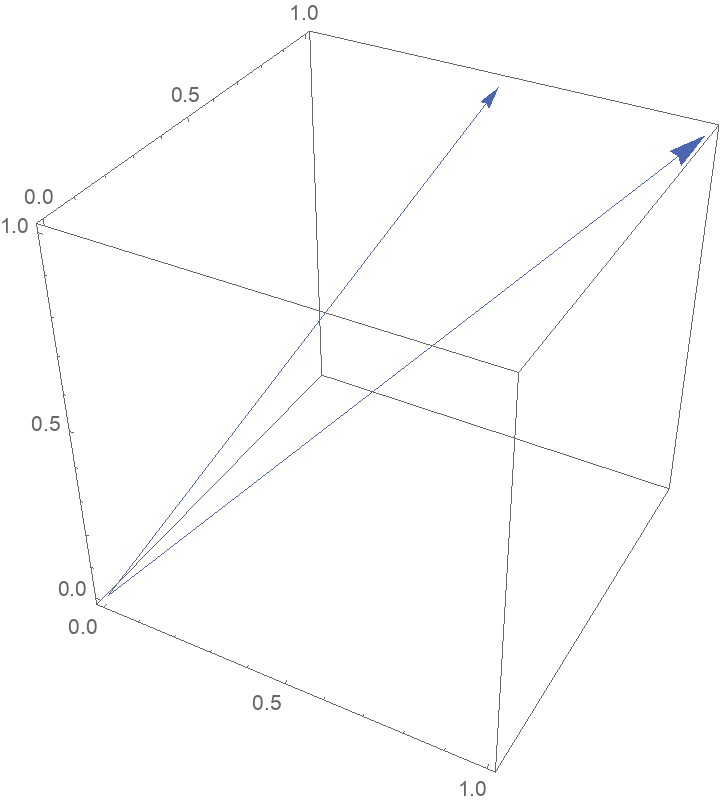#### LocatedVector

A located vector from the first point to the second point:

 In:=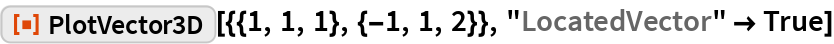Out=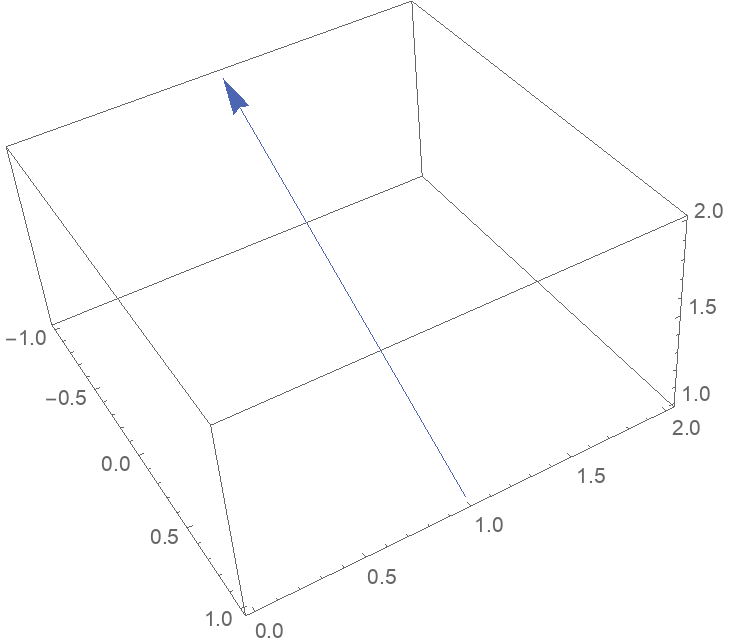Setting "LocatedVector" to False draws the vector located at the origin:

 In:=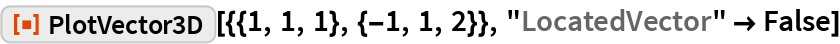Out=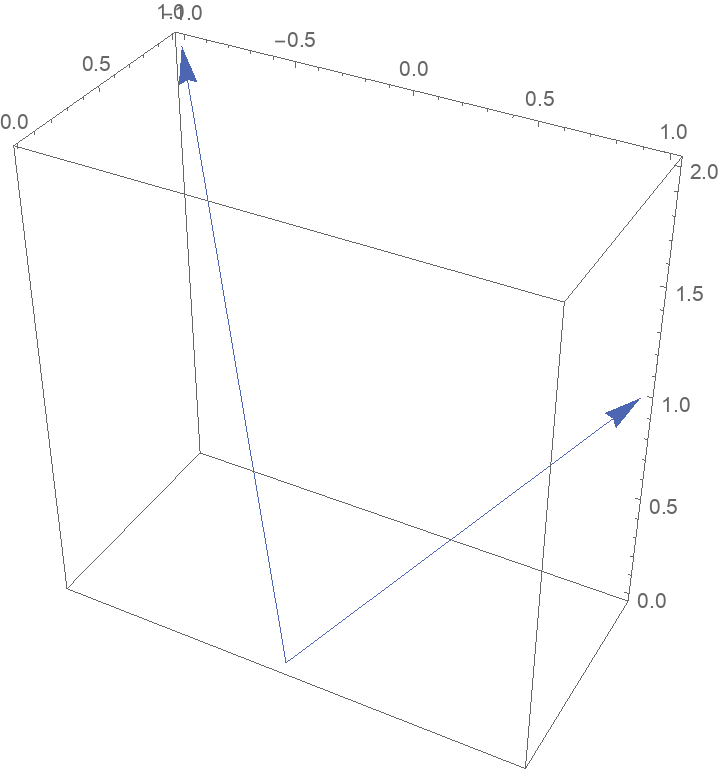A list of lists of pairs of vectors with the option “LocatedVector”->True draws located vectors from the first point in each sublist to the second point in each sublist:

 In:=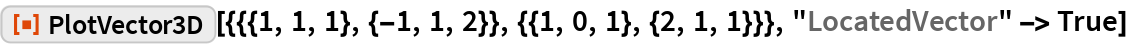Out=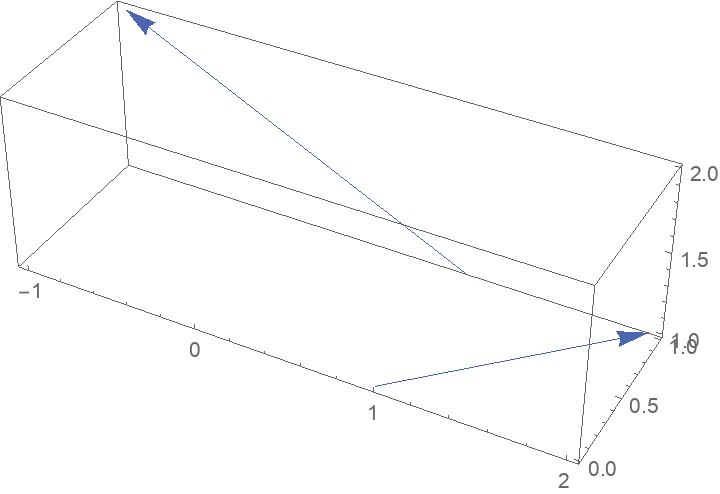Without the option being set, the second tuple in each list gives the located point, and the first tuple gives the direction:

 In:=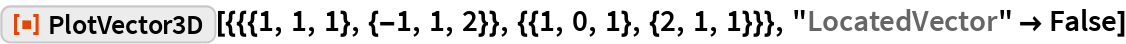Out=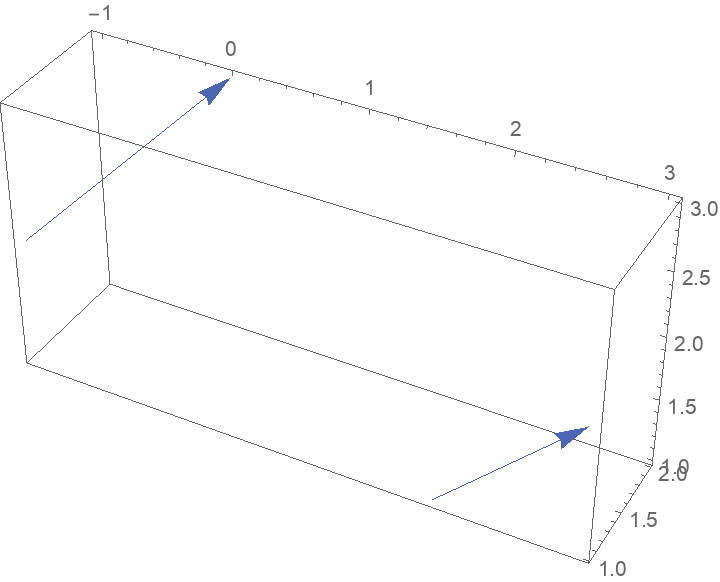#### VectorStyle

Change the style of the vectors:

 In:=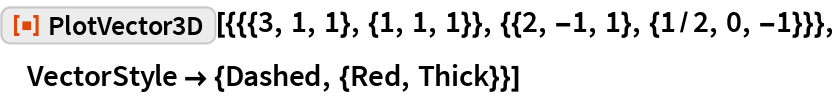Out=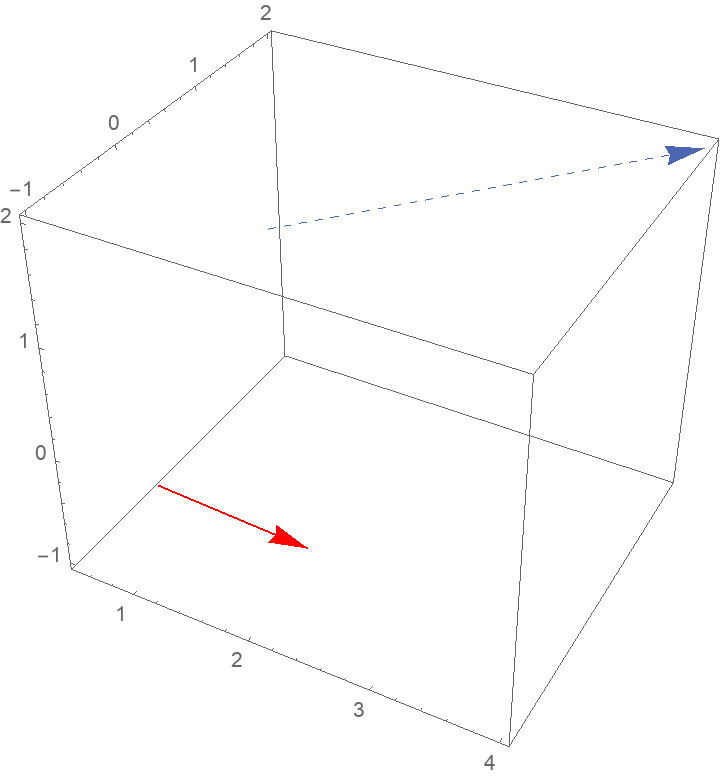Set both the arrow size and style for each vector:

 In:=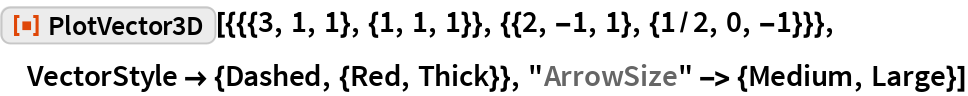Out=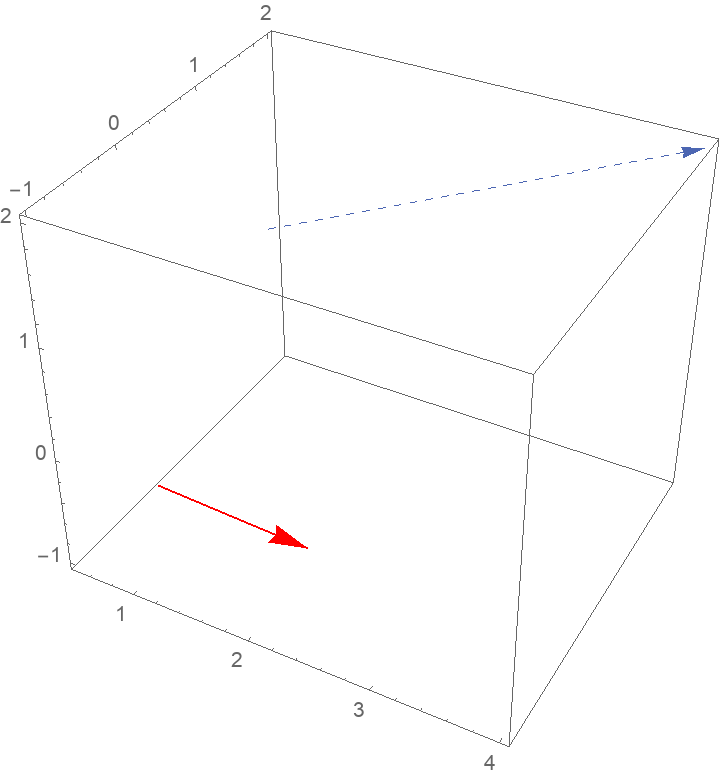### Properties and Relations

You can specify a vector located at a point using the 2nd, 3rd and 4th templates:

 In:=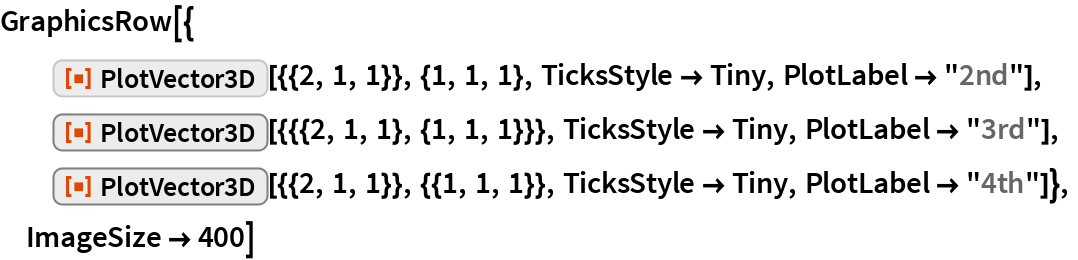Out=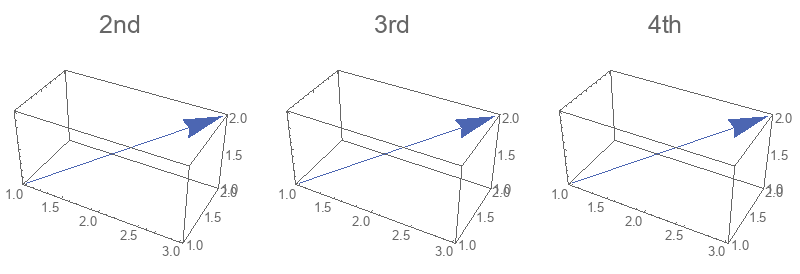Use Arrow to produce any of these plots:

 In:=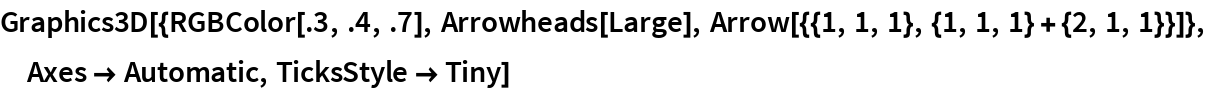Out=